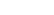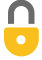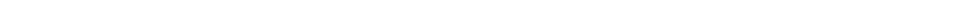Problem 18
Q

# Determine the conversion factor between

1. km/h and mi/h,
2. m/s and ft/s, and
3. km/h and m/s.A
a) 0.6214 mi/hr
b) 3.28 ft/s
c) 0.278 m/s Note at 2:03 I mistakenly crossed out the m in meters when I meant to circle the meters. Thank you @schabs6 for pointing this out in the comments.In order to watch this solution you need to have a subscription.VIDEO TRANSCRIPT

This is Giancoli Answers with Mr. Dychko. For converting kilometers per hour into miles per hour, we need to create a fraction that has the unit we don't want being canceled away and this fraction has to introduce the unit that we do want so what we want here in our answer is we want miles per hour so that means we need this fraction to introduce miles on the top and we need it to get rid of kilometers and so we need to know how many miles there are per kilometer and the front cover of the textbook tells us it's 0.6214 miles per kilometer and sure enough the kilometers cancel because they are on opposite sides of the fraction here and we are left with miles per hour. So 1 kilometer per hour is going to end up being 0.6214 miles per hour but this is our conversion factor right here— 0.6214 miles per kilometer is our conversion factor. And then in the second case, we wanna be left with feet per second so this conversion factor is chosen so that the feet unit is introduced on the top and that the meter unit will be canceled away and so we have 3.28 feet per meter and that's our conversion factor here. And converting kilometers per hour into meters per second, our final answer has to have meters on top and seconds on the bottom. So we introduce a few fractions to achieve that. So for one thing, we'll get rid of the hour time unit by multiplying by 1 hour for every 60 minutes but that doesn't quite get us all the way because we need to get rid of the minutes then and convert that into seconds. So we have the hours canceling and the minutes canceling and we'll be left with seconds now on the bottom so that's good and then we need to take care of the distance unit and so get rid of kilometers and introduce meters. And if you multiply this whole thing out, you will get 0.277 and however many significant figures you need for based on whatever quantity you are working with— maybe 0.278. And then the unit for our conversion factor— this will make meters per second by the way— but as a final answer for our conversion factor, probably be better to say 0.278 hour meters per kilometer second. So let me show you how that comes about. So we are looking just at this conversion factor part here just whatever's in here. And when you work just in units within that, you see that the minutes cancel and you are left with hour meters on top and kilometer seconds on the bottom. So conversion factor is 0.278 hour meters per kilometer second and whenever that meets some number expressed in kilometers per hour, you will see that the hours cancel and so do the kilometers; you are left with meters per second.